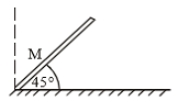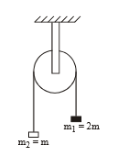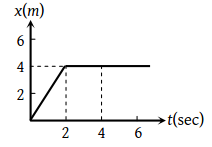A hollow sphere is rolling without slipping on the inclined plane. The minimum coefficient of friction is :

1. $\frac{2}{5}\mathrm{tan}\theta$

2. $\frac{2}{3}\mathrm{tan}\theta$

3. $\frac{3}{4}\mathrm{tan}\theta$

4. $\frac{5}{7}\mathrm{tan}\theta$

Concept Questions :-

Rolling motion
High Yielding Test Series + Question Bank - NEET 2020

Difficulty Level:

A body of mass (m) is projected with velocity v0 at an angle of projection $\mathrm{\theta }$. The magnitude of angular momentum about origin after time t from projection is :

1. Increase with time

2. decrease with time

3. independent of time

4. none of these

Concept Questions :-

Angular momentum
High Yielding Test Series + Question Bank - NEET 2020

Difficulty Level:

A body of mass m slides down an incline and reaches the bottom with a velocity v. If the same mass were in the form of a ring which rolls down this incline, the velocity of the ring at bottom would have been

(1) v

(2)

(3) $\left(1/\sqrt{2}\right)v$

(4) $\left(\sqrt{2/5}\right)v$

Concept Questions :-

Rolling motion
High Yielding Test Series + Question Bank - NEET 2020

Difficulty Level:

A rod is released at an angle 45$°$ to the horizontal as shown. If its length is L, its angular acceleration about its foot at ground is initially1. $\frac{3\mathrm{g}}{2\mathrm{L}}$

2. $\frac{3\mathrm{g}}{\sqrt{2}\mathrm{L}}$

3. $\frac{3\mathrm{g}}{2\sqrt{2}\mathrm{L}}$

4. $\frac{6\mathrm{g}}{\sqrt{2}\mathrm{L}}$

Concept Questions :-

Torque
High Yielding Test Series + Question Bank - NEET 2020

Difficulty Level:

From a disc of mass M and radius '2a', a concentric disc of radius 'a' is cut out. The moment of inertia of remaining disc is

1.

2.

3.

4.  $\frac{3}{2}{\mathrm{Ma}}^{2}$

High Yielding Test Series + Question Bank - NEET 2020

Difficulty Level:

The moment of inertia of pulley is I and its radius is R. The string doesn't slips on the pulley, as system is released, the acceleration of the system is(1) $\frac{g}{\left(3+\frac{I}{m{R}^{2}}\right)}$

(2) $\frac{g}{\left(1+\frac{I}{m{R}^{2}}\right)}$

(3) $\frac{3g}{\left(1+\frac{I}{m{R}^{2}}\right)}$

(4) $\frac{3g}{\left(2+\frac{I}{m{R}^{2}}\right)}$

Concept Questions :-

Torque
High Yielding Test Series + Question Bank - NEET 2020

Difficulty Level:

A 100 g iron ball having velocity 10 m/s collides with a wall at an angle 30° and rebounds with the same angle. If the period of contact between the ball and wall is 0.1 second, then the force experienced by the wall is

(1) 10 N

(2) 100 N

(3) 1.0 N

(4) 0.1 N

Concept Questions :-

Linear momentum
High Yielding Test Series + Question Bank - NEET 2020

Difficulty Level:

A man fires a bullet of mass 200 g at a speed of 5 m/s. The gun is of one kg mass. By what velocity the gun rebounds backwards ?

(1) 0.1 m/s

(2) 10 m/s

(3) 1 m/s

(4) 0.01 m/s

Concept Questions :-

Linear momentum
High Yielding Test Series + Question Bank - NEET 2020

Difficulty Level:

A body of mass M at rest explodes into three pieces, two of which of mass M/4 each are thrown off in perpendicular directions with velocities of 3 m/s and 4 m/s respectively. The third piece will be thrown off with a velocity of

(1) 1.5 m/s

(2) 2.0 m/s

(3) 2.5 m/s

(4) 3.0 m/s

Concept Questions :-

Linear momentum
High Yielding Test Series + Question Bank - NEET 2020

Difficulty Level:

In the figure given below, the position-time graph of a particle of mass 0.1 Kg is shown. The impulse at t = 2 sec is(1) 0.2 kg m sec–1

(2) –0.2 kg m sec–1

(3) 0.1 kg m sec–1

(4) –0.4 kg m sec–1

Concept Questions :-

Linear momentum